## SPM

Pencil.my has every feature you will ever need
to study by yourself.
We’re Here to
Make you A Star!

Upon completion of lower secondary education, students have the opportunity to pursue two years of study in upper secondary (Form 4 and Form 5).

At the end of Form 5, students are required to take the Sijil Pelajaran Malaysia (SPM) or Malaysian Certificate of Education before graduating their secondary school.

The subjects taken in the SPM exam are:
• Bahasa Melayu
• Bahasa Inggeris (English)
• Science
• Mathematics
• Pendidikan Islam
• Sejarah
• Biology (Elective Subject)
• Physics (Elective Subject)
• Chemistry (Elective Subject)
• Prinsip Perakaunan (Elective Subject)
• Perdagangan (Elective subject)
• Ekonomi Asas (Elective Subject)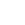### Bahasa Melayu

Bahasa Melayu consists of two papers – Bahasa Melayu Paper 1 and Paper 2. Both papers are writing papers. Candidates are given 2 hours 15 minutes to complete Bahasa Melayu Paper 1 and 2 hours 30 minutes for Bahasa Melayu Paper 2.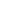### Bahasa Inggeris (English)

English consists of two papers – English Paper 1 and English Paper 2. Paper 1 is writing paper and candidates are required to answer all the questions in 1 hour 45 minutes.
Paper 2 consists for sections – Section A (multiple choice question), Section B (fill in the blanks), Section C (structured question), and Section D (English Literature Component). The time given to complete this paper is 2 hours 15 minutes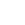### Mathematics

Mathematics for SPM consists of two papers, Mathematics Paper 1 and Mathematics Paper 2. Paper 1 consists of 40 multiple questions with the time given 1 hour 15 minutes whereas for Paper 2, it is the structured question which consists of 2 sections, Section A and Section B. Students are compulsory to show calculation on paper. The time given to complete Paper 2 is 2 hours 30 minutes. Formulae are given and candidates are allowed to use calculator during examination.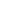Additional Mathematics for SPM consists of two papers, Additional Mathematics Paper 1 and Additional Mathematics Paper 2. Both papers writing papers where students need to show calculation on the papers. Additional Mathematics Paper 1 need to be completed in 2 hours while Additional Mathematics Paper 2 need to be completed in 2 hours 30 minutes. Formula are given and candidates are allowed to use calculator during examination.### Sejarah

Sejarah SPM consists of 3 papers. Paper 1 is the multiple choice question and it consists of 40 questions to be completed in 1 hour. Paper 2 is the structured questions paper. Sejarah Paper 2 consists of two section, Section A and Section B. Candidates are required to answer all question in Section A and 3 questions in Section B. The time given to complete Paper 2 is 2 hours and 30 minutes.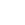### Pendidikan Islam

Pendidikan Islam SPM consists of two papers, Paper 1 and Paper 2. Pendidikan Islam Paper 1 consists of two sections, section A dan Section B. Section A consists of 20 multiple choice question and 20 fill in the blank questions while section B is the writing section. Candidates are required to answer three questions for section B. The total time given to complete Paper 1 is 2 hours. Paper 2 is a structured question paper. Candidates are required to answer all the questions in 1 hour and 40 minutes.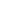### Prinsip Perakaunan (Elective Subject)

Prinsip Perakaunan consists of two papers – Paper 1 and Paper 2. Paper is the multiple choice question and candidates need to complete the paper in 1 hour 15 minutes.
Paper 2 is the calculation paper. Candidates are required to show all the solution and calculation and it need to be completed in 2 hours 30 minutes.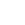### Biology (Elective Subject)

Biology consists of three papers, Biology Paper 1, Paper 2 and Paper 3. Paper 1 consists of 50 multiple choice questions and need to be completed in 1 hours 15 minutes.
Paper 2 and Paper 3 are both writing papers. Paper 2 consists of 2 section, Section A and Section B. Candidates are required to answer all the questions in section A and two question is section B. Time given to complete this paper is 1 hour 15 minutes.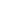### Chemistry (Elective Subject)

Chemistry for SPM consists of 3 papers – Chemistry Paper 1, Paper 2 and Paper 3. Paper 1 consists of 50 multiple choice question and the time given to complete this paper is 1 hour 15 minutes.
Paper 2 is a structured question paper. There are three sections in this paper – Section A, Section B and C. Candidates are required to answer all questions in section A and 1 question in both section B and C. The paper need to be completed in 2 hours and 30 minutes.
Paper 3 is a writing paper. It focus more on the experiment part. Candidates are required to answer all the questions in 1 hour and 30 minutes.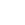### Physics (Elective Subject)

Physics consists of 3 papers – Physics Paper 1, Paper 2 and Paper 3. Paper 1 consists of 50 multiple choice questions and the time given to complete the paper is 1 hour and 15 minutes.
Paper 2 is a structured question paper. There are three sections in this paper – Section A, Section B and C. Candidates are required to answer all questions in section A and 1 question in both section B and C. The paper need to be completed in 2 hours and 30 minutes.
Paper 3 is a writing paper. It focus more on the experiment part. There are two sections in the paper – Section A and Section B. Candidates are required to answer all the questions in section A and one question in Section B, and need to be completed in 1 hour and 30 minutes.### Ekonomi Asas (Elective Subject)

Ekonomi Asas consists of two papers – Paper 1 and Paper 2. Paper is the multiple choice question and candidates need to complete the paper in 1 hour 15 minutes. Paper 2 is the writing paper. This paper consists of two sections – Section A and Section B. Candidates are required to answer 3 question in Section A and 1 question in Section B. Time given to complete the paper is 2 hours.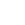### Perdagangan (Elective Subject)

Perdagangan consists of two papers – Paper 1 and Paper 2. Paper is the multiple choice question and candidates need to complete the paper in 1 hour 15 minutes.
Paper 2 is the writing paper. This paper consists of two sections – Section A and Section B. Candidates are required to answer 3 question in Section A and 1 question in Section B. Time given to complete the paper is 2 hours.